# Quantum Chemistry Quantum Mechanics Density Functional Theory Background

• Slides: 26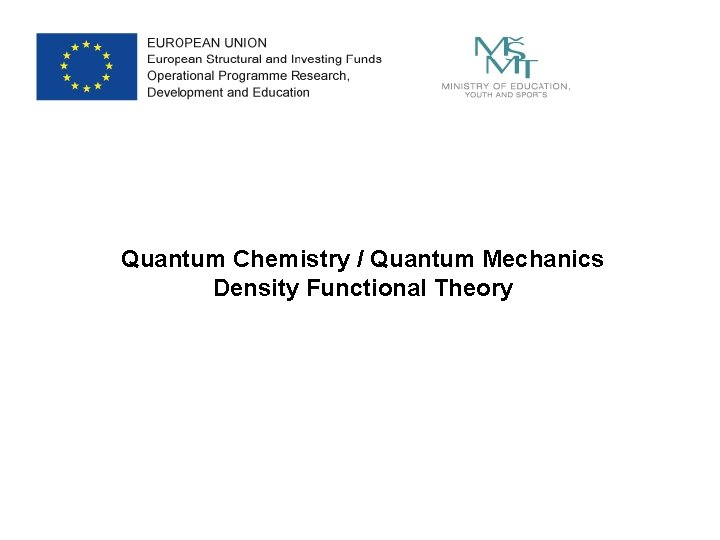Quantum Chemistry / Quantum Mechanics Density Functional Theory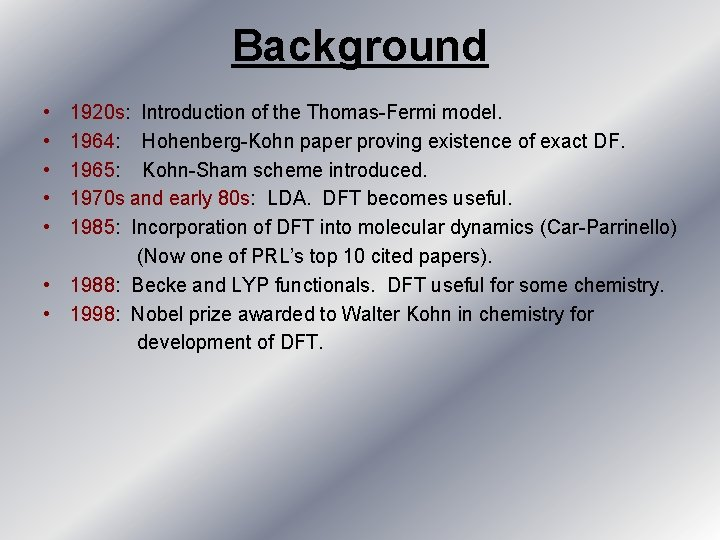Background • • • 1920 s: Introduction of the Thomas-Fermi model. 1964: Hohenberg-Kohn paper proving existence of exact DF. 1965: Kohn-Sham scheme introduced. 1970 s and early 80 s: LDA. DFT becomes useful. 1985: Incorporation of DFT into molecular dynamics (Car-Parrinello) (Now one of PRL’s top 10 cited papers). • 1988: Becke and LYP functionals. DFT useful for some chemistry. • 1998: Nobel prize awarded to Walter Kohn in chemistry for development of DFT.External Potential: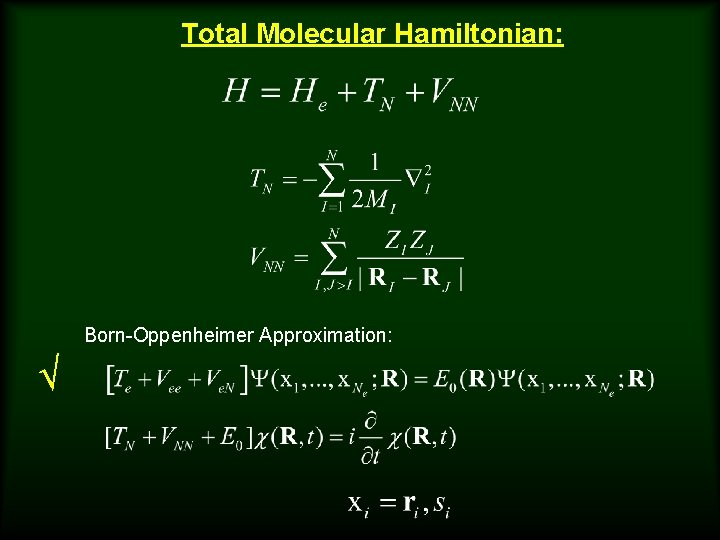Total Molecular Hamiltonian: Born-Oppenheimer Approximation: √Hohenberg-Kohn Theorem • Two systems with the same number Ne of electrons have the same Te + Vee. Hence, they are distinguished only by Ven. • Knowledge of |Ψ 0> determines Ven. • Let V be the set of external potentials such solution of yields a non=degenerate ground state |Ψ 0>. Collect all such ground state wavefunctions into a set Ψ. Each element of this set is associated with a Hamiltonian determined by the external potential. There exists a 1: 1 mapping C such that C: V ΨHohenberg-Kohn Theorem (part II) Given an antisymmetric ground state wavefunction from the set Ψ, the ground-state density is given by Knowledge of n(r) is sufficient to determine |Ψ> Let N be the set of ground state densities obtained from Ne-electron ground state wavefunctions in Ψ. Then, there exists a 1: 1 mapping D: Ψ N D-1 : N Ψ The formula for n(r) shows that D exists, however, showing that D-1 exists Is less trivial.The energy functional The energy expectation value is of particular importance From the variational principle, for |Ψ> in Ψ: Thus, Therefore, E[n 0] can be determined by a minimization procedure: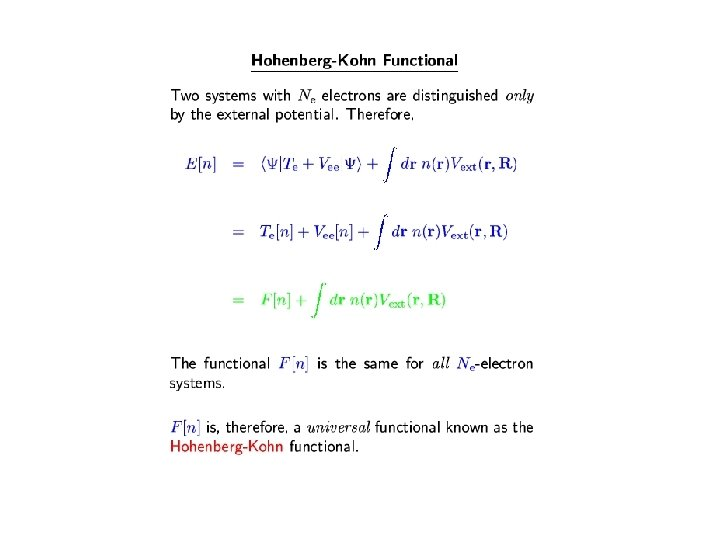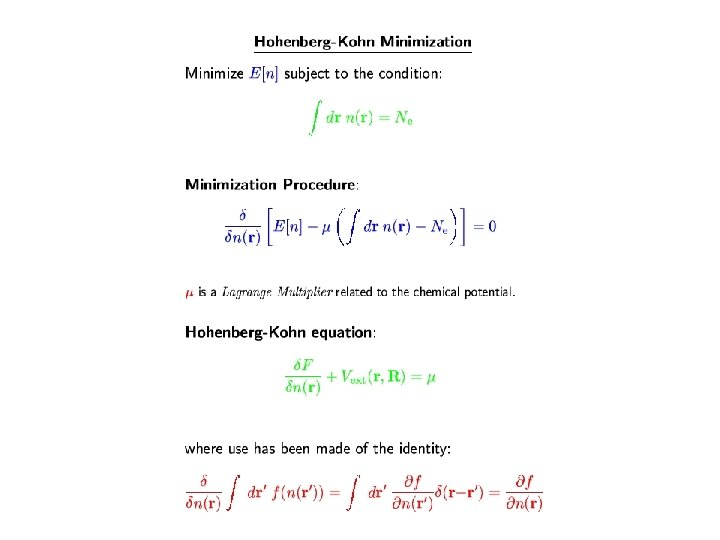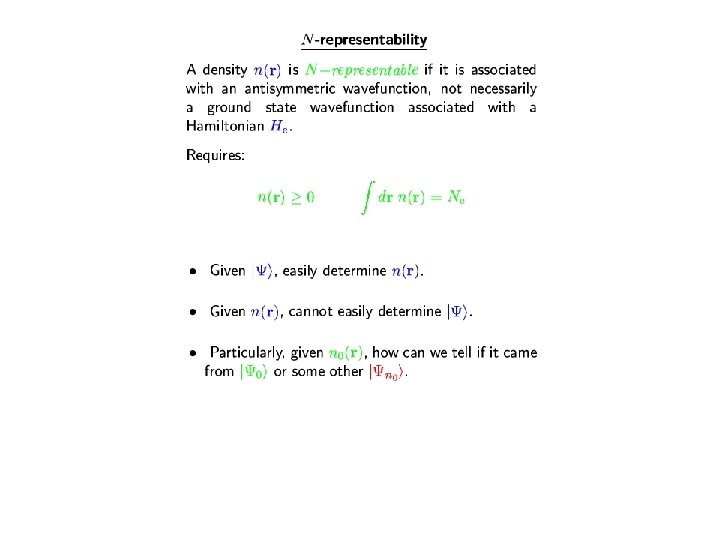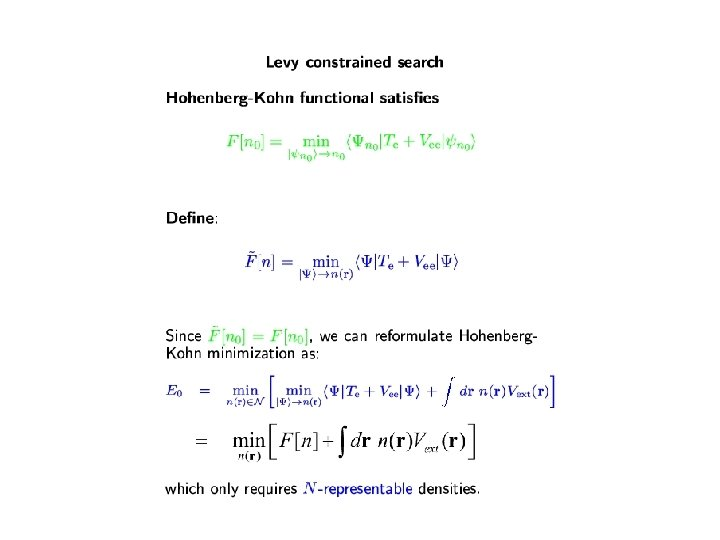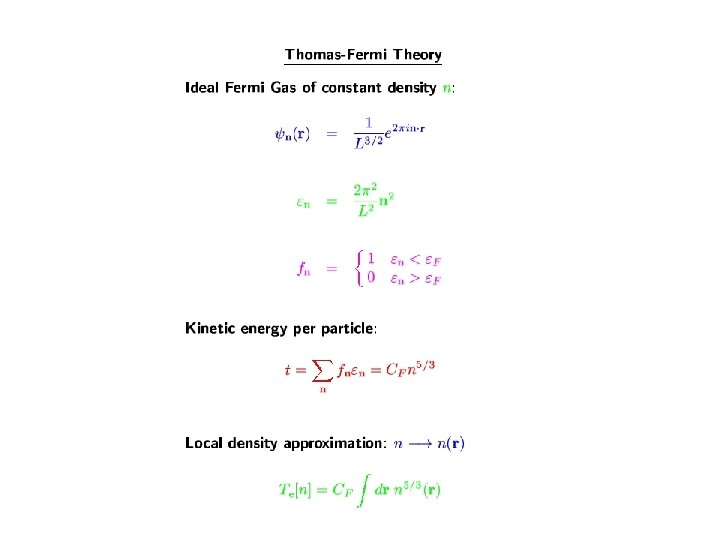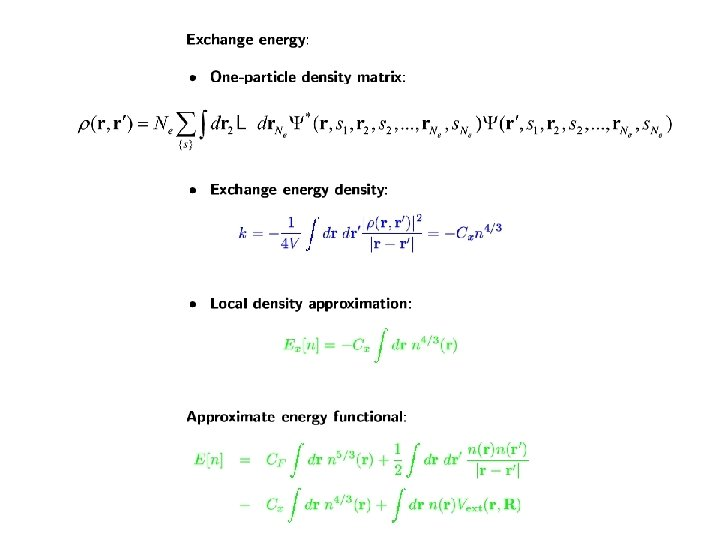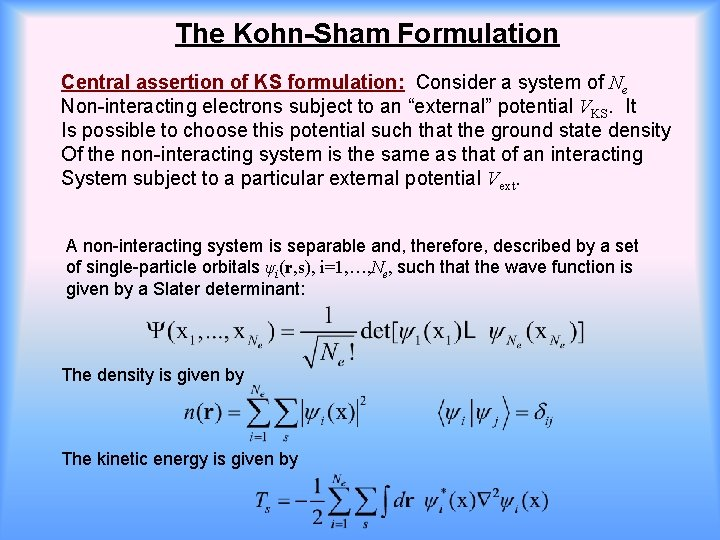The Kohn-Sham Formulation Central assertion of KS formulation: Consider a system of Ne Non-interacting electrons subject to an “external” potential VKS. It Is possible to choose this potential such that the ground state density Of the non-interacting system is the same as that of an interacting System subject to a particular external potential Vext. A non-interacting system is separable and, therefore, described by a set of single-particle orbitals ψi(r, s), i=1, …, Ne, such that the wave function is given by a Slater determinant: The density is given by The kinetic energy is given by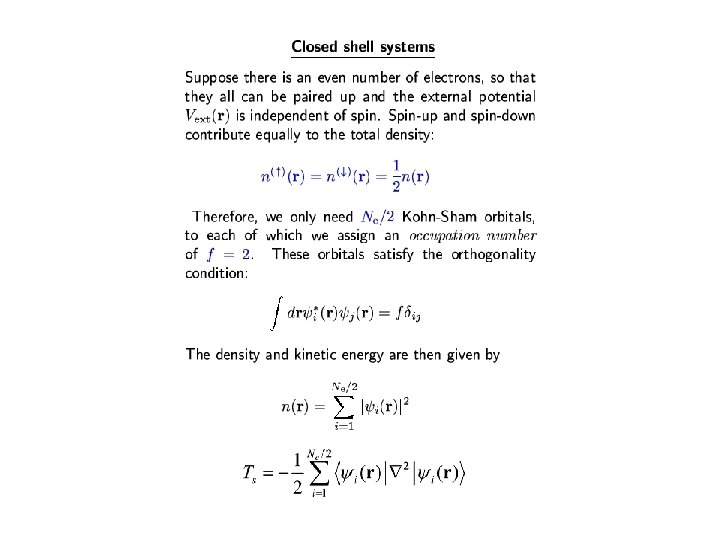Some simple results from DFT Ebarrier(DFT) = 3. 6 kcal/mol Ebarrier(MP 4) = 4. 1 kcal/mol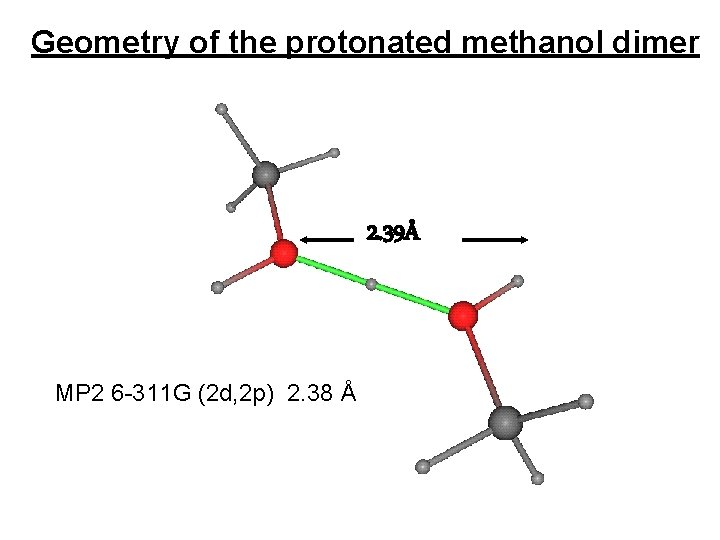Geometry of the protonated methanol dimer 2. 39Å MP 2 6 -311 G (2 d, 2 p) 2. 38 ÅResults methanol Dimer dissociation curve of a neutral dimer Expt. : -3. 2 kcal/mol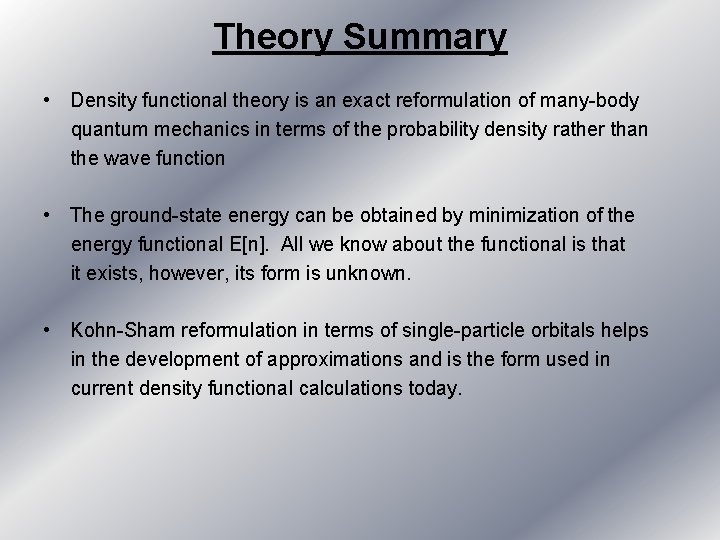Theory Summary • Density functional theory is an exact reformulation of many-body quantum mechanics in terms of the probability density rather than the wave function • The ground-state energy can be obtained by minimization of the energy functional E[n]. All we know about the functional is that it exists, however, its form is unknown. • Kohn-Sham reformulation in terms of single-particle orbitals helps in the development of approximations and is the form used in current density functional calculations today.Functional Overview CPU time for energy local V( ) eg. Fermi-Dirac pure V( , , ) non-local, GGA V( , , ) eg. LSDA, BPW 91 t ~ N 3 functional types hybrid V( , , ) + VHF t ~ N 4 no dispersion eg. B 3 LYP with dispersion/ correction eg. ωB 97 XD „double hybrid“ V( , , ) + VMP 2 eg. m. PW 2 PLYP t ~ N 5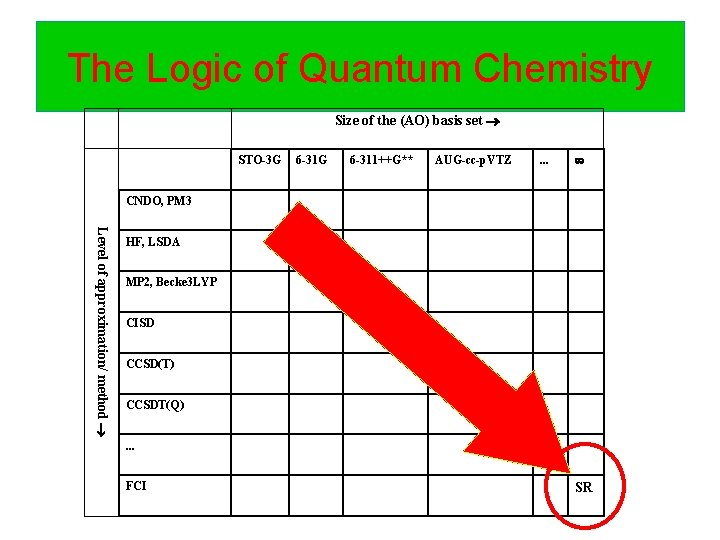The Logic of Quantum Chemistry Size of the (AO) basis set STO-3 G 6 -311++G** AUG-cc-p. VTZ . . . CNDO, PM 3 Level of approximation/ method HF, LSDA MP 2, Becke 3 LYP CISD CCSD(T) CCSDT(Q). . . FCI SR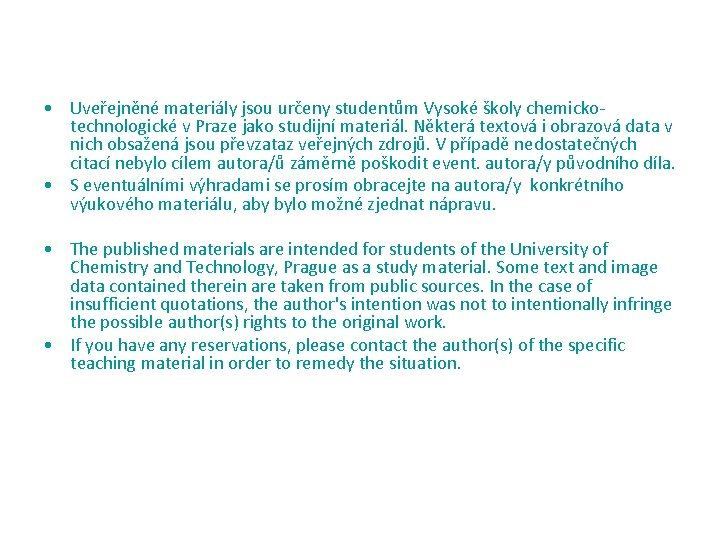• Uveřejněné materiály jsou určeny studentům Vysoké školy chemickotechnologické v Praze jako studijní materiál. Některá textová i obrazová data v nich obsažená jsou převzataz veřejných zdrojů. V případě nedostatečných citací nebylo cílem autora/ů záměrně poškodit event. autora/y původního díla. • S eventuálními výhradami se prosím obracejte na autora/y konkrétního výukového materiálu, aby bylo možné zjednat nápravu. • The published materials are intended for students of the University of Chemistry and Technology, Prague as a study material. Some text and image data contained therein are taken from public sources. In the case of insufficient quotations, the author's intention was not to intentionally infringe the possible author(s) rights to the original work. • If you have any reservations, please contact the author(s) of the specific teaching material in order to remedy the situation.